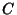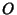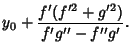Letbe a curve and letbe a fixed point. Letbe onand letbe the Curvature Center at. Letbe the point witha line segment Parallel and of equal length to. Then the curve traced byis the radial curve of. It was studied by Robert Tucker in 1864. The parametric equations of a curvewith Radial PointareCurve Radial Curve Astroid Quadrifolium Catenary Kampyle of Eudoxus Cycloid Circle Deltoid Trifolium Logarithmic Spiral Logarithmic Spiral Tractrix Kappa Curve

References

Lawrence, J. D. A Catalog of Special Plane Curves. New York: Dover, pp. 40 and 202, 1972.

Yates, R. C. Radial Curves.'' A Handbook on Curves and Their Properties. Ann Arbor, MI: J. W. Edwards, pp. 172-174, 1952.